# Cyclic spanning subdigraph with small cyclomatic number

 Importance: Medium ✭✭
 Subject: Graph Theory » Directed Graphs
 Keywords:
 Posted by: fhavet on: June 2nd, 2013
Conjecture   Let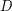be a digraph all of whose strong components are nontrivial. Thencontains a cyclic spanning subdigraph with cyclomatic number at most.

The {\it cyclomatic number} of a digraphis. For a strong digraph, it correspond to the minimum of directed ears in a directed ears decomposition. (See Chapter 5 of [BM]).denotes the {\it stability number} of the digraph, that is the maximum number of pairwise non-adjacent vertices.

Bessy and Thomassé [BT04] showed that any nontrivial strong digraphhas a spanning subdigraph which is the union ofdirected cycles. However, the structure of this subdigraph might be rather complicated. This leads one to ask whether there always exists a spanning subdigraph whose structure is relatively simple, one which is easily seen to be the union ofdirected cycles. A natural candidate would be a spanning subdigraph built from a directed cycle by addingdirected ears. But or any, there exists a digraphwith stability numberrequiring at leastdirected ears. See Chapter 19 of [BM08].

A possible way around this problem is to allow spanning subdigraphs which are disjoint union of strong digraphs. Such digraph are called cyclic (because each arc lies on a directed cycle). The conjecture was formulated by Bondy[B], based on a remark of Chen and Manalastas [CM].

The Conjecture holds forby Camion's Theorem [C] and also for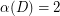and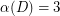by theorems of Chen and Manalastas [CM] and S. Thomassé (unpublished), respectively.

The conjecture implies not only the above-mentioned Bessy--Thomassé Theorem, but also a result of Thomassé [Thom01], that the vertex set of any strong digraphwith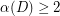can be partitioned intodirected paths, as well as another theorem of Bessy and Thomassé [BT03], that every strong digraphhas a strong spanning subdigraph with at most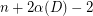arcs.

## Bibliography

[BT03] S. Bessy and S. Thomassé, Every strong digraph has a spanning strong subgraph with at most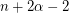arcs. J. Combin. Theory Ser. B 87 (2003), 289--299.

[BT04] S. Bessy and S. Thomassé, Three min-max theorems concerning cyclic orders of strong digraphs. In Integer Programming and Combinatorial Optimization, 132--138. Lecture Notes in Comput. Sci., Vol. 3064, Springer, Berlin.

*[B95] J.A. Bondy, Basic graph theory: paths and circuits. In Handbook of Combinatorics, Vol. 1, 3--110. Elsevier, Amsterdam.

[BM] J.A. Bondy and U.S.R. Murty, Graph Theory, volume 244 of Graduate Texts in Mathematics. Springer, 2008.

[C] P. Camion, Chemins et circuits hamiltoniens des graphes complets. C. R. Acad. Sci. Paris 249 (1959), 2151--2152.

[CM] C.C. Chen C.C. and Jr. P. Manalastas, Every finite strongly connected digraph of stability 2 has a Hamiltonian path. Discrete Math. 44 (1983), 243--250.

[T] S. Thomassé, Covering a strong digraph by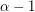disjoint paths: a proof of Las Vergnas' conjecture. J. Combin. Theory Ser. B 83 (2001), 331--333.

* indicates original appearance(s) of problem.